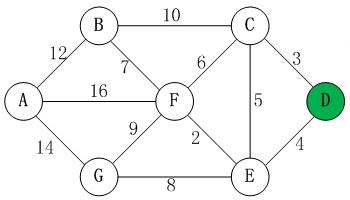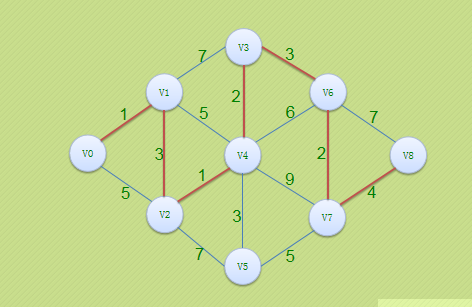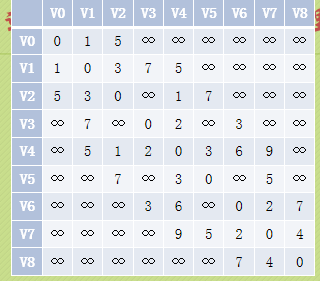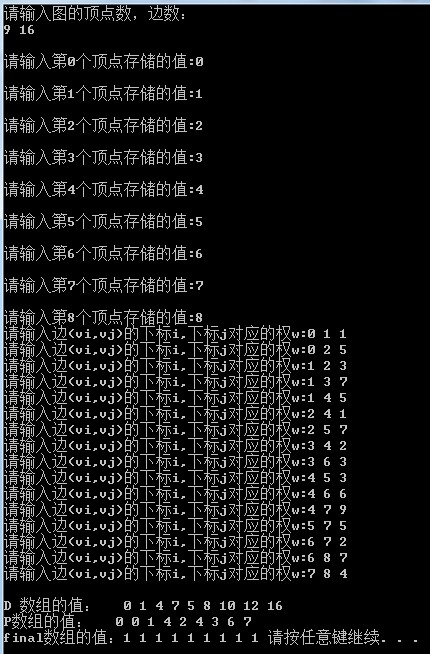• 迪杰斯特拉算法C语言实现)
2022-04-15 20:38:59#include<stdio.h>
#include<string.h>
#include<math.h>

//给定有向/无向加权图G(V, E)，给定源点/起始点s，求从s出发到V中其它所有顶点的权重最小的路径。(迪杰斯特拉算法描述)
/*
算法思路：
1.逐步地发展最短路径树，直至它覆盖所有顶点。
2.构造一个循环，每次循环都增加一个顶点到最短路径树上。
3.从所有与树邻接的顶点中，选择离源点最近的。
4.对每个顶点，都用一个距离标记(Label)来记录。
5.每次循环都需要对距离标记进行更新。

*/
int main(){
//使用邻接矩阵构建整个图，其中权重代表传播时延（都是int type），使用INT_MAX(被证明不行，因为加了就溢出了）所以用65535代替，代表图中两个顶点不相连
int g = {
65535,12,65535,65535,65535,16,14,
12,65535,10,65535,65535,7,65535,
65535,10,65535,3,5,6,65535,
65535,65535,3,65535,4,65535,65535,
65535,65535,5,4,65535,2,8,
16,7,6,65535,2,65535,9,
14,65535,65535,65535,8,9,65535
};
//定义一维距离数组表示源点到图中所有点的距离，将被不断更新(其更新过程十分类似BFS)
int distance={0};
//定义已选择数组，用于表示该点已被选择(1)，在教程图中代表列表S，未被选择的在教程图中代表列表U
int selected={0};
//这里选择源点A;      //修改单源顶点修改start即可，后面已实现解耦写法
int start=3;
selected[start]=1;
int index={0}; //用于表示顶点被选择的顺序
int l=0;
index[l++]=start;
//至此，需要建立的存储结构结束，开始初始化distance数组（读取邻接矩阵）
for(int i=0;i<7;i++){
distance[i]=g[start][i];
}
//构建一个循环，在列表U中，选出一个离源顶点最近的顶点
for(int a=0;a<6;a++){
int min=0;
for(int j=1;j<7;j++){
if(selected[j]==0&&distance[j]<distance[min]){ //g[start][min]这个写法应该有点问题
min=j;
}
}
selected[min]=1; //最小距离的被选出
index[l++]=min;
for(int k=0;k<7;k++){ //根据被选出的顶点更新distance数组
if(selected[k]==0&&distance[k]>distance[min]+g[min][k]){ //因为目前的最小生成树发生变化，以新选出的结点来更新到其他还没有加入到最短生成树的节点的最短路径，可根据看图来编写代码
distance[k]=distance[min]+g[min][k];
}
}
}
printf("依次选出的顶点为 ");
for(int b=0;b<7;b++){
printf("%c ",'A'+index[b]); //通过ASCII码字符表示连续的原理来显示 'A'到'B'只需要'A'+1即可
}
printf("\n");
for(int c=0;c<7;c++){
printf("源点D到点%c的距离为%d\n",'A'+c,distance[c]); //懒得解耦这个输出格式为start
}
return 0;
}c++
更多相关内容
• 迪杰斯特拉算法算法步骤： （1）初始时，S只包含源点。 （2）从U中选取一个距离v最小的顶点k加入S中（该选定的距离就是v到k的最短路径长度）。 （3）以k为新考虑的中间点，修改U中各顶点的距离；若从源点v到...
• 一、 迪杰斯特拉算法思想 Dijkstra算法主要针对的是有向图的单元最短路径问题，且不能出现权值为负的情况！Dijkstra算法类似于贪心算法，其应用根本在于最短路径的最优子结构性质。 最短路径的最优子结构性质： ...
• 迪杰斯特拉算法c语言实现简介测试用例，一个无向图源码测试结果 简介 原理：路径长度依次递增原理。 辅助向量： D[]:记录带权长度。每一轮都在向小的值或者不变的值更新。 P[]用来存放前驱顶点。 final[]用来存放某...

### 简介

原理：路径长度依次递增原理。
辅助向量：
D[]:记录带权长度。每一轮都在向小的值或者不变的值更新。
P[]用来存放前驱顶点。
final[]用来存放某顶点是否已经进入S（已经求得最短路径的顶点集合）集合。

### 测试用例，一个无向图其邻接矩阵图：### 源码


/************************
*最短路径之dijkstra
*D[]用来存放带权长度。每一轮都在向小的值或者不变的值更新。
*P[]用来存放前驱顶点
*final[]用来存放某顶点是否已经进入S（已经求得V0到Vw的最短路径。）集合。final[w]=1表示已经求得顶点V0到Vw的最短路径 ，即w已经进入S集合了。
******************************************************/

#include<stdio.h>
#include<stdlib.h>
#define MAXVER 100  //最大顶点数
#define INFINITY 65535 //65535表示无穷大（邻接表中 权为无穷大表示没有弧）

typedef	int	Patharc[MAXVER];			// 用于存储最短路径下标的数组
typedef int	ShortPathTable[MAXVER];		// 用于存储到各点最短路径的权值和

typedef struct  MGraph
{
char vertex[MAXVER] ;  //顶点表
int arc[MAXVER][MAXVER] ;//邻接矩阵
int numVertexes, numEdges;//图中当前顶点数和边数
}MGraph;

//建立无向网的邻接矩阵
void  CreateGraph(MGraph *G)
{
int i, j,k,w;
//设置顶点个数
printf("请输入图的顶点数，边数：\n");
scanf("%d %d", &G->numVertexes, &G->numEdges);
//getchar();//清空缓冲区（主要是回车）
setbuf(stdin, NULL);//设置输入缓冲区 为空缓冲区

//设置结点存储值
for (i = 0; i < G->numVertexes; i++)
{
printf("\n请输入第%d个顶点存储的值:",i);
scanf("%c", &G->vertex[i]);

// printf("%c",G->vertex[i]);

setbuf(stdin, NULL);//设置输入缓冲区 为空缓冲区（每次输入一个回车，这里会造成 \n字符存在缓冲区）
}
for (i = 0; i < G->numVertexes; i++)
{
for (j = 0; j < G->numVertexes; j++)
{
if(i == j)
{
G->arc[i][j] = 0;
}
else
{
G->arc[i][j] = INFINITY;//初始化邻接矩阵（权为INFINITY无穷大表示没有弧）
}
}
}
for (k = 0; k < G->numEdges; k++)
{
printf("请输入边(vi,vj)的下标i,下标j对应的权w:");
scanf("%d %d %d", &i, &j, &w);
G->arc[i][j] = w;//设置对应的权
G->arc[j][i] = G->arc[i][j];//无向网，对称矩阵
}
}

void ShortestPath_Dijkstra(MGraph G ,int V0,Patharc *P,ShortPathTable *D)
{
int  v,w,k,min;
int final[MAXVER]; //final[w]=1表示已经求得顶点V0到Vw的最短路径 ，即w已经进入S集合了。

//初始化数据
for(v=0;v<G.numVertexes;v++)
{
final[v]=0;
(*D)[v] = G.arc[V0][v];
(*P)[v] = 0;
}
(*D)[V0] = 0; //V0到V0的路径为0
final[V0] = 1; //V0到V0不需要求路径

//开始主循环，每次求得V0到某个V顶点的最短路径。
for(v=1 ;v<G.numVertexes;v++)
{
min =INFINITY;
for(w=0;w<G.numVertexes;w++)
{
if(!final[w]  && (*D)[w] <min)
{
k = w;
min = (*D)[w];
}
}
final[k] = 1; // 将目前找到的最近顶点置1

//修正当前最短路径及距离。
for(w=0;w<G.numVertexes;w++)
{
//如果经过V顶点的路径比现在这条路径的长度短的话，更新!
if(!final[w]  && min+G.arc[k][w]<(*D)[w])
{
(*D)[w] = min+G.arc[k][w]; //修改当前路径长度
(*P)[w] = k;  // 存放前驱结点。
}
}
}

//输出数组D，P,final
printf("\nD 数组的值：");
for(v=0 ;v<G.numVertexes;v++)
{
printf("%d ",(*D)[v]);
}
printf("\nP数组的值：");
for(v=0 ;v<G.numVertexes;v++)
{
printf("%d ",(*P)[v]);
}
printf("\nfinal数组的值：");
for(v=0 ;v<G.numVertexes;v++)
{
printf("%d ",final[v]);
}
}

int main()
{
MGraph G;
CreateGraph(&G);
Patharc P[MAXVER];
ShortPathTable D[MAXVER];

ShortestPath_Dijkstra(G,0,P,D);

system("pause");
return 0;
}


### 测试结果展开全文数据结构

# 算法思想

\quad 设置两个顶点集合 S S T T ，集合 S S 中存放已经找到的最短路径的顶点，集合 T T 中存放当前还未找到的路径的顶点。初始状态时，集合 S S 中只包含源点，设为 v 0 {v_0} ，然后从集合 T T 中选择找到源点 v 0 {v_0} 路径长度最短的顶点 u u 加入到集合 S S 中，集合 S S 中每加入一个新的顶点 u {u} ，都要修改源点 v 0 {v_0} 到集合T中剩余的顶点的当前的最短路径长度值，集合 T T 中各顶点新的当前最短路径长度值为原来的当前最短路径长度值与源点过顶点 到达该顶点的路径长度的较小者。此过程不断重复，直到集合 T T 中所有的顶点全部加入到集合 S S 中为止。

# 函数模块

（ 1 ） （1） 该程序主要包含四个头文件，分别为 A d j M G r a p h . h , A d j M G r a p h C r e a t . h , D i j s t r a . h , S e q L i s t . h 。 AdjMGraph.h,AdjMGraphCreat.h,Dijstra.h,SeqList.h。
（ 2 ） D i j s t r a . h （2） Dijstra.h 中存放 D i j s t r a ( A d j M G r a p h G , i n t v 0 , i n t d i s t a n c e [ ] , i n t p a t h [ ] ) Dijstra(AdjMGraph G,int v0,int distance[ ],int path[]) 。该函数共有四个参数，两个输入参数，分别为带权图 G G ,源点序号 v 0 {v_0} ;连个输出参数分别为 d i s t a n c e [ ] distance[] p a t h [ ] path[]

# 数据结构

## 图

t y p e d e f s t r u c t typedef struct
{
S e q L i s t V e r t i c e s ; / / 存 放 顶 点 的 顺 序 表 SeqList Vertices;//存放顶点的顺序表
i n t e d g e [ M a x V e r t i c e s ] [ M a x V e r t i c e s ] ; / / 存 放 边 的 邻 接 矩 阵 int edge[MaxVertices][MaxVertices];//存放边的邻接矩阵
i n t n u m O f E d g e s ; / / 边 的 条 数 int numOfEdges;//边的条数
} A d j M G r a p h ; AdjMGraph;

## 带权图

t y p e d e f s t r u c t typedef struct
{
i n t r o w ; / / 行 int row;//行
i n t c o l ; / / 列 int col;//列
i n t w e i g h t ; / / 权 值 int weight;//权值
} R o w C o l W e i g h t ; RowColWeight;

# 测试数据

（ 1 ） （1） 顶点集合 A , B , C , D , E , F {A,B,C,D,E,F}
（ 2 ） （2） 边的集合{{ 0 , 2 , 5 0,2,5 },{ 0 , 3 , 25 0,3,25 },{ 1 , 0 , 4 1,0,4 },{ 1 , 4 , 6 1,4,6 },{ 2 , 1 , 15 2,1,15 },{ 2 , 5 , 9 2,5,9 },{ 4 , 3 , 8 4,3,8 },{ 5 , 3 , 12 5,3,12 },{ 5 , 4 , 20 5,4,20 }}

# C语言程序

## 头文件

### A d j M G r a p h . h AdjMGraph.h

#ifndef ADJMGRAPH_H_INCLUDED

#include"SeqList.h"
typedef struct
{
SeqList Vertices;//存放顶点的顺序表
int edge[MaxVertices][MaxVertices];//存放边的邻接矩阵
int numOfEdges;//边的条数

{
int i,j;
for(i=0;i<n;i++)
for(j=0;j<n;j++)
{
if(i==j)
G->edge[i][j]=0;//不存在自环
else
G->edge[i][j]=MaxWeight;//边的权值为无穷大，即不存在边
}
G->numOfEdges=0;//初始时边的数量为0
ListInitiate(&G->Vertices);
}

{
ListInsert(&G->Vertices,G->Vertices.size,vertex);//在顺序表尾部插入
//顺序表，插入位置，数据元素
}

void InsertEdge(AdjMGraph *G,int v1,int v2,int weight)
//在图G插入边<v1,v2>,权值为weight
{
if(v1<0||v1>=G->Vertices.size||v2<0||v2>=G->Vertices.size)
{
printf("插入时，参数v1或v2不合法\n");
return;//函数结束标志
}
if(0<G->edge[v1][v2] && G->edge[v1][v2]<weight)//边已存在，不能覆盖
{
printf("边已存在\n");
return;//函数结束标志
}
G->edge[v1][v2]=weight;
G->numOfEdges++;
}

{
if(v1<0||v1>=G->Vertices.size||v2<0||v2>=G->Vertices.size||v1==v2)
{
printf("删除时，参数v1或v2不合法\n");
return;//函数结束标志
}
if(G->edge[v1][v2]==MaxWeight||v1==v2)
{
printf("该边不存在，无法删除!\n");
return;
}
G->edge[v1][v2]=MaxWeight;
G->numOfEdges--;
}

//在图G中寻找序号v的顶点的第一个邻接顶点
{
int col;
if(v<0||v>=G.Vertices.size)
{
printf("参数出界，获取失败!\n");
return -1;
}
for(col=0;col<G.Vertices.size;col++)
if(G.edge[v][col]>0 && G.edge[v][col]<MaxWeight)
return col;
return -1;//未找到
}

//在图中找v1顶点的邻接顶点v2的下一个顶点
{
int col;
if(v1<0||v1>=G.Vertices.size||v2<0||v2>=G.Vertices.size)
{
printf("参数v1或v2越界，获取失败!\n");
return -1;//函数结束标志
}
for(col=v2+1;col<G.Vertices.size;col++)
if(G.edge[v1][col]>0 && G.edge[v1][col]<MaxWeight)
return col;
return -1;//未找到
}



### A d j M G r a p h C r e a t . h AdjMGraphCreat.h

#ifndef ADJMGRAPHCREAT_H_INCLUDED

typedef struct
{
int row;//行
int col;//列
int weight;//权值
}RowColWeight;

void CreatGraph(AdjMGraph *G,DataType V[],int n,RowColWeight E[],int e)
//在图中插入n个顶点信息V和e条边信息E,n是顶点数，e是边数
{
int i,k;
Initiate(G,n);//顶点顺序表初始化
for(i=0;i<n;i++)//插入顶点
InsertVertex(G,V[i]);
for(k=0;k<e;k++)//插入边
InsertEdge(G,E[k].row,E[k].col,E[k].weight);
}



### D i j s t r a . h Dijstra.h

#ifndef DIJKSTRA_H_INCLUDED
#define DIJKSTRA_H_INCLUDED

#endif // DIJKSTRA_H_INCLUDED
void Dijkstra(AdjMGraph G, int v0, int distance[], int path[])
//带权图G从下标v0顶点到其他顶点的最短距离distance和最短路径下标path
{
int n=G.Vertices.size;//
int *s=(int *)malloc(sizeof(int )*n);
int minDis,i,j,u;
//初始化
for(i=0;i<n;i++)
{
distance[i]=G.edge[v0][i];
s[i]=0;//标记
if(i!=v0 && distance[i]<MaxWeight)
path[i]=v0;
else
path[i]=-1;
}
s[v0]=1;//标记顶点v0已从集合T加入到集合S中
//当前还未找到最短路径的顶点集合中选取具有最短路径的顶点u
for(i=1;i<n;i++)
{

minDis=MaxWeight;
for(j=0;j<n;j++)
{
if(s[j]==0 && distance[j]<minDis)
{
u=j;
minDis=distance[j];
}
}
//当已不存在路径时，算法结束。此语句对于非连通图是必须的
if (minDis==MaxWeight)return ;//结束程序
s[u]=1;//标记顶点u已从集合T加入到集合S中
//修改从v0到其他顶点的最短路径和最短距离
for(j=0;j<n;j++)
if(s[j]==0 && G.edge[u][j]<MaxWeight && distance[u]+G.edge[u][j]<distance[j])
//松弛操作
{
//顶点v0经过顶点u到其他顶点的最短距离和最短路径
distance[j]=distance[u]+G.edge[u][j];
path[j]=u;

}
}
}



### S e q L i s t . h SeqList.h

#ifndef SEQLIST_H_INCLUDED
#define SEQLIST_H_INCLUDED
#endif // SEQLIST_H_INCLUDED
//typedef  int DataType;//定义DataType为int
typedef struct//线性表定义
{
DataType List[MaxSize];//一维数组
int size;//储存线性表长度
}SeqList;//线性表的名字SeqList，即定义的结构体名称为SeqList

void ListInitiate(SeqList *L)//初始化线性表
{//初始化时，会修改size的值，因此必须用指针
L->size=0;//初试元素个数为0
}
int  ListLength(SeqList L)//线性表元素个数
{//可以不用指针，因为没有修改值，只是返回值
return L.size;
}
int ListInsert(SeqList *L,int i,DataType x)
{//将DataType类型的x插入到线性表L的第i个位置
int j;
if (L->size>=MaxSize)
{
printf("操作不合法，线性表已满！\n");
return 0;
}
else if (i<0||i>L->size)
{
printf("参数不合法！\n");
return 0;
}
else
{
//从后向前依次后移数据，若有10个元素,size=10,则从List开始
for (j=L->size;j>i;j--)
L->List[j]=L->List[j-1];
L->List[i]=x;
L->size++;
return 1;
}
}
int ListDelete(SeqList *L,int i,DataType *x)
{
int j;
if (L->size<=0)
{
printf("操作不合法，线性表已空！\n");
return 0;
}
else if (i>L->size-1||i<0)
{//数组从Lst开始，到Lst[size-1]结束
printf("参数不合法！\n");
return 0;
}
else
{
*x=L->List[i];//保存被删除元素
for(j=i+1;j<=L->size-1;j++)
L->List[j-1]=L->List[j];
L->size--;
return 1;
}

}
int ListGet(SeqList L,int i,DataType *x)
{
if(i<0||i>L.size)
{
printf("参数不合法！\n");
return 0;
}
else
{
*x=L.List[i];
return 1;
}
}



## 主文件

#include <stdio.h>
#include <stdlib.h>
#include<malloc.h>
typedef  char DataType;
#define MaxVertices 10
#define MaxWeight 10000
#define MaxSize 10// 顺序表的长度
#include"Dijkstra.h"
#include''SeqList.h''
int main()
{
char a[]={'A','B','C','D','E','F'};
RowColWeight rcw[]={{0,2,5},{0,3,25},{1,0,4},{1,4,6},{2,1,15},{2,5,9},{4,3,8},{5,3,12},{5,4,20}}
int i,n=6,e=9;
int distance,path;
CreatGraph(&g,a,n,rcw,e);
Dijkstra(g,0,distance,path);
printf("从顶点%c到其他各顶点的最短距离为：\n",g.Vertices.List);
for(i=0;i<n;i++)
printf("到顶点%c的最短距离为%d：\n",g.Vertices.List[i],distance[i]);
printf("从顶点%c到其他各顶点的最短路径的前一顶点为：\n",g.Vertices.List);
for(i=0;i<n;i++)
if(path[i]!=-1)
printf("到顶点%c的前一顶点为%c\n",g.Vertices.List[i],g.Vertices.List[path[i]]);
return 0;
}



# 实验结果展开全文数据结构
• 这个ZIP包含了最短路径算法中两个经典的算法迪杰斯特拉和弗洛伊德），这个是我在大一的时候写的程序设计课程的作业之一。有需要的小伙伴可以下载学习。
• } } void ShortestPath_DIJ(AMGraph G,int v0)//用Dijkstra算法求有向图G的v0到其余顶点的最短路径 { int i,n,v,min,w;//下面所用到的所有变量 n = G.vexnum;//顶点个数 bool S[n];//辅助数组S[]的定义 int D...

还是按照书上的例子：完整代码如下：

#include <stdio.h>
#include <stdlib.h>
#include <string.h>
#define MaxInt 32767//无穷值设置
#define MVNum 100 //图的最大容量 ，也可以称为图的最大顶点数

void Interrupt(void)//创建一个中断函数
{
while(1)//用于检测换行符，使函数脱离scanf的连续输出
if(getchar()=='\n')
break;
}

typedef struct//图的结构体
{
char vexs[MVNum];//顶点表
int arcs[MVNum][MVNum];//邻接矩阵
int vexnum,arcnum;//图的当前点数和边数
}AMGraph;

void InitGraph(AMGraph &G)//图的初始化
{
int i,j;
for(i = 0;i<MVNum;i++)
for(j = 0;j<MVNum;j++)
G.arcs[i][j] = MaxInt;//使邻接矩阵中的数据都初始化为0
}

void CreateGraph(AMGraph &G)//图的创建
{
int i;//记录次数
char a;//顶点变量
printf("请输入顶点数和边数:");
scanf("%d %d",&G.vexnum,&G.arcnum);
Interrupt();//该函数用于检测并吸收换行符
printf("请输入顶点名称（连续输入）：");
for(i=0;i<G.vexnum;i++)
{
scanf("%c",&a);
G.vexs[i] = a;//第i个顶点的命名
}
Interrupt();//该函数用于检测并吸收换行符
char b,c;//顶点变量
int w,j,k;//w为权值变量，j和k是用来记录次数的
for(i=0;i<G.arcnum;i++)
{
printf("请输入边的两个顶点和权值w:");
scanf("%c %c %d",&b,&c,&w);//输入
Interrupt();//该函数用于检测并吸收换行符
for(j=0;j<G.vexnum;j++)
{
if(G.vexs[j] == b)//找到输入的顶点b的位置
break;
}
for(k=0;k<G.vexnum;k++)
{
if(G.vexs[k] == c)//找到输入的顶点c的位置
break;
}
G.arcs[j][k] = w;//权值赋值
}
}

void InputGraph(AMGraph G)//邻接矩阵的输出
{
int i,j;//记录次数
printf("邻接矩阵为：\n   ");
for(i=0;i<G.vexnum;i++)//打印顶点名称
printf("%c  ",G.vexs[i]);
printf("\n");
for(i=0;i<G.vexnum;i++)
{
printf("%c  ",G.vexs[i]);//打印顶点名称
for(j=0;j<G.vexnum;j++)//遍历邻接矩阵
printf("%d  ",G.arcs[i][j]);
printf("\n");
}
}

void ShortestPath_DIJ(AMGraph G,int v0)//用Dijkstra算法求有向图G的v0到其余顶点的最短路径
{
int i,n,v,min,w;//下面所用到的所有变量
n = G.vexnum;//顶点个数
bool S[n];//辅助数组S[]的定义
int D[n],Path[n];//辅助数组D[]和Path[]的定义
for(v=0;v<n;v++)//辅助数组的初始化
{
S[v] = false;
D[v] = G.arcs[v0][v];
if(D[v]<MaxInt)
Path[v] = v0;
else
Path[v] = -1;
}
S[v0] = true;
D[v0] = 0;//初始化结束
for(i=1;i<n;i++)//对其余n-1个顶点，依次进行计算
{
min = MaxInt;//暂定最小权值
for(w=0;w<n;w++)
if(!S[w] && D[w]<min)
{
v = w;
min = D[w];//选择一条从v0出发的最短路径，终点为v
}
S[v] = true;//用于记录已选择过
for(w=0;w<n;w++)//更新从v0出发到集合V-S上所有顶点的最短路径长度
if(!S[w] && (D[v]+G.arcs[v][w]<D[w]))
{
D[w] = D[v]+G.arcs[v][w];//更新D[w]
Path[w] = v;//更新w的前驱为v
}
}
//打印辅助数组最后结果
for(i=0;i<n;i++)//打印D[]
printf("D[%d]=%d  ",i,D[i]);
printf("\n");
for(i=0;i<n;i++)//打印Path
printf("Path[%d]=%d  ",i,Path[i]);
printf("\n");
}

int main()
{
AMGraph G;
InitGraph(G);//图的初始化
CreateGraph(G);//创建邻接矩阵
InputGraph(G);//输出邻接矩阵
ShortestPath_DIJ(G,0);//Dijkstra算法，数字0可换成变量，这里是测试
return 0;
}



结果演示：
辅助数组的初始化结果代码运行结果（完）

展开全文数据结构 最短路径
• Dijkstra算法 Dijkstra(迪杰斯特拉)算法是典型的最短路径路由算法，是广度优先算法的一种，用于计算一个节点到其他所有节点的最短路径。主要特点是以起始点为中心向外层层扩展，直到扩展到终点为止。其基本原理是：...
•数据结构 开发语言
• Dijkstra（迪杰斯特拉）算法是典型的最短路径路由算法，用于计算一个节点到其他所有节点的最短路径。...下面这篇文章就给大家介绍关于C++用Dijkstra算法(迪杰斯特拉算法)求最短路径的方法，下面来一起看看吧。
• C语言实现迪杰斯特拉算法，亲测好用，放在正确的环境下就可运行
• 迪杰斯特拉是单源最短路算法(即只能求一点，到其他任一点的最短路径，但可以加循环得到任意两点间的最短路径)，无法处理带负权变的图 算法思路图示 初始化两个集合 S={A}(只包含源点，表示已经确定最短路径的节点，...
• 自己用c语言写的迪杰斯特拉算法，存储方式为邻接矩阵表示法
•数据结构
• 本文参考自《大话数据结构》，网上有很多关于迪杰斯特拉和弗洛伊德算法的描述和图解，大家都可以去看看，这里就不赘述，只是对迪杰斯特拉算法做简单实现。 在网图和非网图中，最短路径的含义是不同的。由于非网图它...数据结构 动态规划
• 最近做软设，地铁换乘管理系统，需要用到迪杰斯特拉算法。正好又复习了一遍，顺便把实现代码作为自己的第一篇博客。 #include #include #define INF 1000000 #define MAX 100 typedef struct Graph{ int vexnum;//...
• 关于Dijkstra(迪杰斯特拉)算法的总结，两种存储结构上C语言实现数据结构
• 文章目录1.找到初始顶点X到各个顶点的权值（这里X为A）第2步和第3步用一个for(i1.找到初始顶点X到各个顶点的权值（这里X为A） for (int i = 0; i < n; i++) { dist[i] = GetWeight(g,v,i); ...数据结构
• 有向网（有权值） 存储：邻接矩阵 最短路径：迪杰斯特拉算法 输出部分:邻接矩阵输出，最短路径输出 头 #include #include #define Max 100 //定义顶点最大容量 #define MaxWeight 32767 //表示极大值 //二进制的原码...数据结构
• 单源最短路径算法——Dijkstra算法&lpar;...其基本原理如下: (1)初始化:集合vertex_set初始为{sourc ...Dijkstra【迪杰斯特拉算法】有关最短路径的最后一个算法——Dijkstra 迪杰斯特拉算法是...
• 初学者。如果有误请指正，欢迎联系QQ2684162190 #include<stdio.h> #include<stdlib.h> #define INFIN 65535 #define MAX_VERTEX_NUM 20 int final;...typedef enum{DG,DN,UDG,UDN}GraphKind;...
• 写在前面：博主是一位普普通通的19届二本大学生，平时最大的爱好就是听听歌，逛逛B站。博主很喜欢的一句话花开堪折直须折，莫待无花空折枝：博主的理解是头一次为人，就应该做自己想...求最短路径的两种算法简介 2.迪.
• 先是朴素版的迪杰斯特拉算法 存储结构为邻接矩阵 int dijstra(int n) { for (int i = 1; i <= n; i++) { dist = 0; int index = -1; for (int j = 1; j <= n; j++)//找到已更新结点（dist值被...数据结构
• 1 Dijkstra算法 1.1 描述 1.2 实现方法 1.3 算法流程图 1.4 伪代码 void Dijkstra( graph G,& path,int v0) { float dist[n]; for(i=1;i<=n;i++) { if(A[v0][i] !=∞) { dist[i]=A[v0][i]; ...c++
• = Infinity && (z == Infinity || x+y } //迪杰斯特拉算法 bool CGraph::ShortPath_Dijkatral(VexType v,int distance[]) { int i,j,k,s,t,w,r= Infinity; bool found[MaxVexNum+1]; ArcPtr p; int d[MaxVexNum+1]; ...
• 目的：在一张地图中找出地点A和地点B的一条最短路径（实际上该算法每次运算会求出地点A到其他各个地点的各一条最短路径）。 过程： 1）以从1号地点到4号地点为例。 2）标记1号地点。（标记的作用将在后面得到体会，...c++...